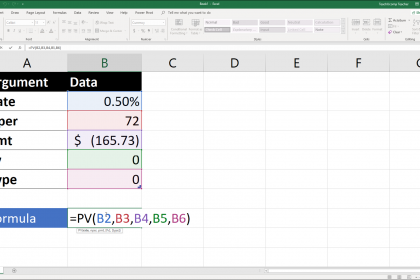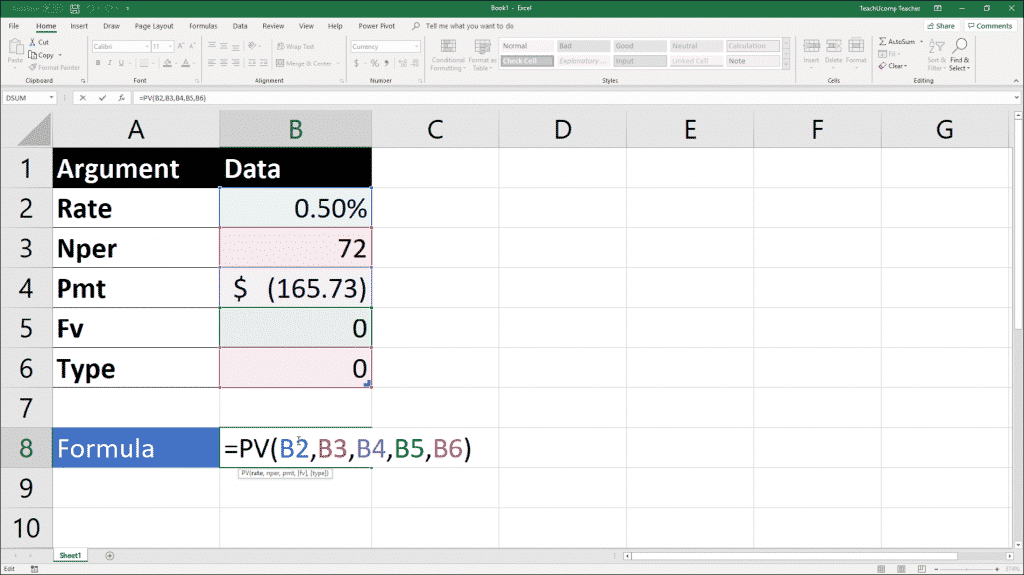# The Pv Function- Excel for Lawyers Tutorial and Video Lesson

/ / Excel 2013, Excel 2016, Excel 2019, Excel for Office 365, Latest, Microsoft, Microsoft Excel Training for Lawyers, Office 2013, Office 2016, Office 2019, Office 365## The Pv Function: Overview

This lesson shows you how to use the Pv function in Excel. Most of the functions that a lawyer needs to know assist them in business and financial computations. You can use these functions to help both the law firm as well as its clients. For example, calculating the future value of a purchased asset is often useful for both parties. While it is often said that it always important to have a calculator available as a lawyer, Excel also doubles as a calculator. Excel is a calculator that contains a vast array of business functions that can assist both you and your clients in many ways.

The first function that we will be learning about is the Pv function. The Pv function assists you in determining the present value of an investment. The present value is the total amount that a series of future payments is worth right now. For example, when you borrow money, the present value to the lender is the original loan amount.###### The Pv Function- Excel for Lawyers Tutorial: A picture of a user creating a formula that uses the Pv function in Excel.

While this may not seem like a function that you would use very often, the Pv function contains many arguments that allow it to be very useful. Note that arguments are simply the pieces of information that a function needs in order to return a result. Let’s examine the syntax of the Pv function and the arguments that the function uses.

 Formula Syntax: PV(rate, nper, pmt, [fv], [type]) Arguments: Rate Required The interest rate per period. For example, if you receive an interest rate of 6% per year on a loan, the monthly rate on the payments would be 6%/12, or .5%. You could enter 6%/12, .5%, or 0.005 into the formula as the Rate argument. Nper Required The total number of payment periods. For example, if you make monthly payments on a 6-year loan, the loan has 72 payments. You would enter 72 as the Nper argument within the formula. Pmt Required The unchanging payment made each period. Typically includes payment and interest, but no fees. On a \$10,000 loan at a 6% annual interest rate for 6 years the payment amount would be \$165.73. You would enter -165.73 into the formula as the pmt argument. Fv Optional The future value or cash balance that you want to attain after the last payment is made. If omitted, Excel assumes this argument is zero. For example, if you wanted to save \$50,000 over 20 years, then enter \$50,000 as the fv argument in the formula. Note that if you omit the fv argument, then the pmt argument is required. Type Optional Enter 0, or omit, if payments are due at the end of the period. Enter 1 if the payments are due at the beginning of each period.

## The Pv Function: Instructions

1. Select the cell in the worksheet where you want the result of the function to appear.
2. Type =PV(rate, nper, pmt, [fv], [type]), where rate, nper, pmt, fv and type are the values, or cell references to cells within the worksheet that contain the values, that you wish to use. Note that the first three are required arguments and the last two are optional.
3. Exit the cell to see the result of the function.

## The Pv Function: Video Lesson

The following video lesson, titled “The Pv Function,” shows how to use the Pv function in Excel. This video lesson is from our complete Excel for Lawyers tutorial, titled “Mastering Excel Made Easy for Lawyers v.2019 and 365.”

TOP
Sale! \$49 All-Access 0 Days 6 Hours 27 Minutes 15 Seconds      \$199 \$49 Entire Library!